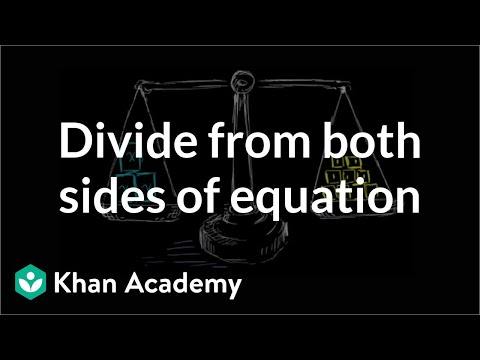Video

# One-step division equations (Full video)

Description: Here's an introduction to basic algebraic equations of the form ax=b. Let's say we have the equation 7 times x is equal to 14. Now before even trying to solve this equation, what I want to do is think a little bit about what this actually means. 7x equals 14, this is the exact same thing as saying 7 times x is equal to 14.

### Other videos you might be interested in### Dividing both sides of an equation (Full video)